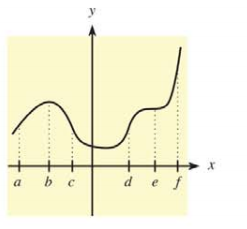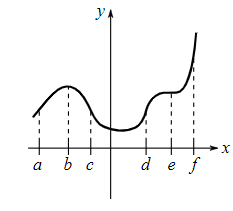Chapter 10.2, Problem 3E### Mathematical Applications for the ...

11th Edition
Ronald J. Harshbarger + 1 other
ISBN: 9781305108042

#### Solutions

Chapter
Section### Mathematical Applications for the ...

11th Edition
Ronald J. Harshbarger + 1 other
ISBN: 9781305108042
Textbook Problem

# In Problems 5-10, use the indicated x-values on the graph of y = f ( x ) to find the following.Find intervals over which the graph is concave down.

To determine

The intervals over which the provided graph of function f(x) is concave down.Explanation

Given Information:

The provided graph is,

Explanation:

Consider the provided graph,

The slope of function f(x) is decreasing in the interval (a,c) and second derivative f(x) of function f(x) is negative in the interval (a,c). Therefore, the graph of function f(x) is concave down on an interval (a,c)

### Still sussing out bartleby?

Check out a sample textbook solution.

See a sample solution

#### The Solution to Your Study Problems

Bartleby provides explanations to thousands of textbook problems written by our experts, many with advanced degrees!

Get Started

#### Divide: (60)(+12)

Elementary Technical Mathematics

#### The implied domain of is: (1, ∞) (−∞, 1) x ≠ 1 (−1, 1)

Study Guide for Stewart's Single Variable Calculus: Early Transcendentals, 8th

#### True or false:

Study Guide for Stewart's Multivariable Calculus, 8th